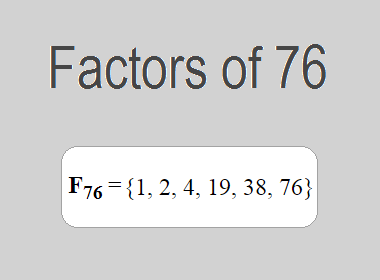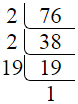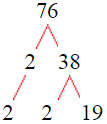# Factors of 76The factors of 76 are 1, 2, 4, 19, 38 and 76 i.e. F76 = {1, 2, 4, 19, 38, 76}. The factors of 76 are all the numbers that can divide 76 without leaving a remainder.

We can check if these numbers are factors of 76 by dividing 76 by each of them. If the result is a whole number, then the number is a factor of 76. Let's do this for each of the numbers listed above:

·        1 is a factor of 76 because 76 divided by 1 is 76.

·        2 is a factor of 76 because 76 divided by 2 is 38.

·        4 is a factor of 76 because 76 divided by 4 is 19.

·        19 is a factor of 76 because 76 divided by 19 is 4.

·        38 is a factor of 76 because 76 divided by 38 is 2.

·        76 is a factor of 76 because 76 divided by 76 is 1.

## How to Find Factors of 76?

1 and the number itself are the factors of every number. So, 1 and 76 are two factors of 76. To find the other factors of 76, we can start by dividing 76 by the numbers between 1 and 76. If we divide 76 by 2, we get a remainder of 0. Therefore, 2 is a factor of 76. If we divide 76 by 3, we get a remainder of 1. Therefore, 3 is not a factor of 76.

Next, we can check if 4 is a factor of 76. If we divide 76 by 4, we get a remainder of 0. Therefore, 4 is also a factor of 76. We can continue this process for all the possible factors of 76.

Through this process, we can find that the factors of 76 are 1, 2, 4, 19, 38 and 76. These are the only numbers that can divide 76 without leaving a remainder.

********************

********************

## Properties of the Factors of 76

The factors of 76 have some interesting properties. One of the properties is that the sum of the factors of 76 is equal to 140. We can see this by adding all the factors of 76 together:

1 + 2 + 4 + 19 + 38 + 76 = 140

Another property of the factors of 76 is that the prime factors of 76 are 2, and 19 only.

## Applications of the Factors of 76

The factors of 76 have several applications in mathematics. One of the applications is in finding the highest common factor (HCF) of two or more numbers. The HCF is the largest factor that two or more numbers have in common. For example, to find the HCF of 76 and 57, we need to find the factors of both numbers and identify the largest factor they have in common. The factors of 76 are 1, 2, 4, 19, 38 and 76. The factors of 57 are 1, 3, 19, and 57. The largest factor that they have in common is 19. Therefore, the HCF of 76 and 57 is 19.

Another application of the factors of 76 is in prime factorization. Prime factorization is the process of expressing a number as the product of its prime factors. The prime factors of 76 are 2, and 19 since these are the only prime numbers that can divide 76 without leaving a remainder. Therefore, we can express 76 as:

76 = 2 × 2 × 19

We can do prime factorization by division and factor tree method also. Here is the prime factorization of 76 by division method,76 = 2 × 2 × 19

Here is the prime factorization of 76 by the factor tree method,76 = 2 × 2 × 19

## Conclusion

The factors of 76 are the numbers that can divide 76 without leaving a remainder. The factors of 76 are 1, 2, 4, 19, 38 and 76. The factors of 76 have some interesting properties, such as having a sum of 140. The factors of 76 have several applications in mathematics, such as finding the highest common factor and prime factorization.